• Slides: 98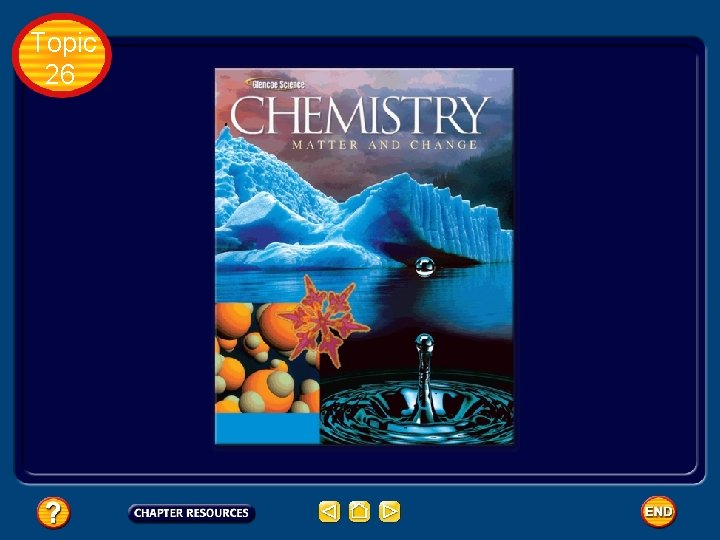Topic 26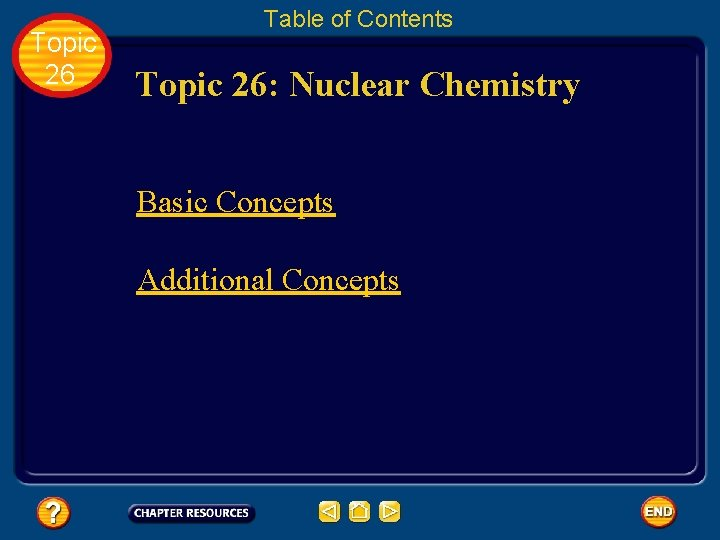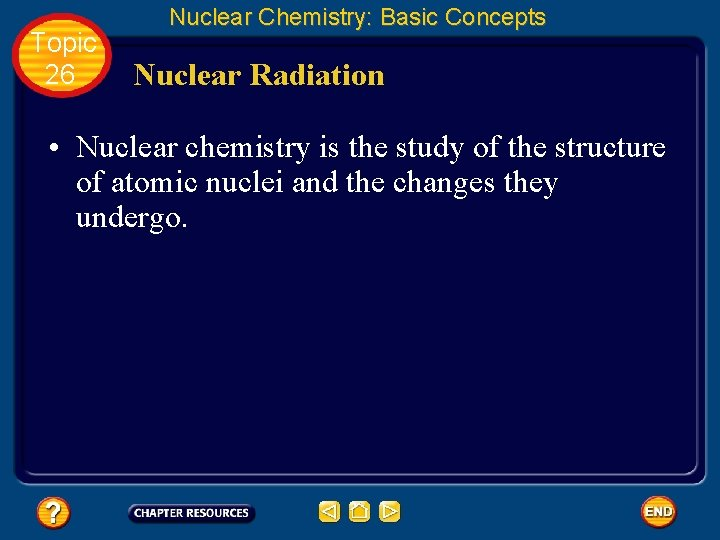Topic 26 Nuclear Chemistry: Basic Concepts Nuclear Radiation • Nuclear chemistry is the study of the structure of atomic nuclei and the changes they undergo.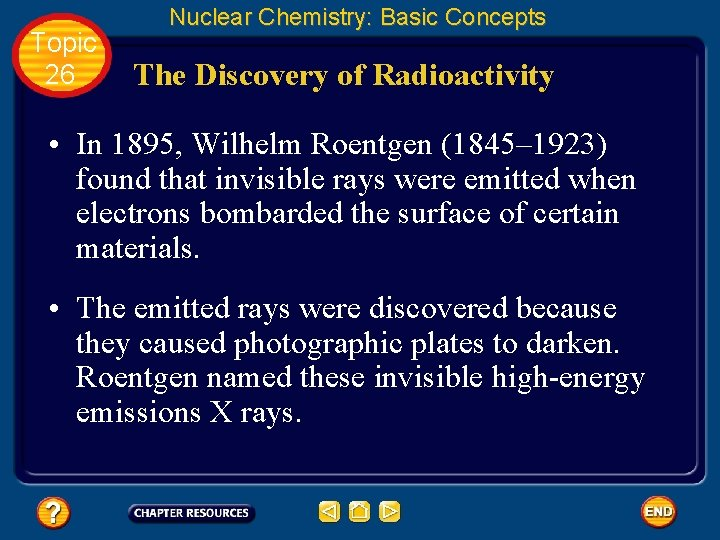Topic 26 Nuclear Chemistry: Basic Concepts The Discovery of Radioactivity • In 1895, Wilhelm Roentgen (1845– 1923) found that invisible rays were emitted when electrons bombarded the surface of certain materials. • The emitted rays were discovered because they caused photographic plates to darken. Roentgen named these invisible high-energy emissions X rays.Topic 26 Nuclear Chemistry: Basic Concepts The Discovery of Radioactivity • As is true in many fields, Roentgen’s discovery of X rays created excitement within the scientific community and stimulated further research.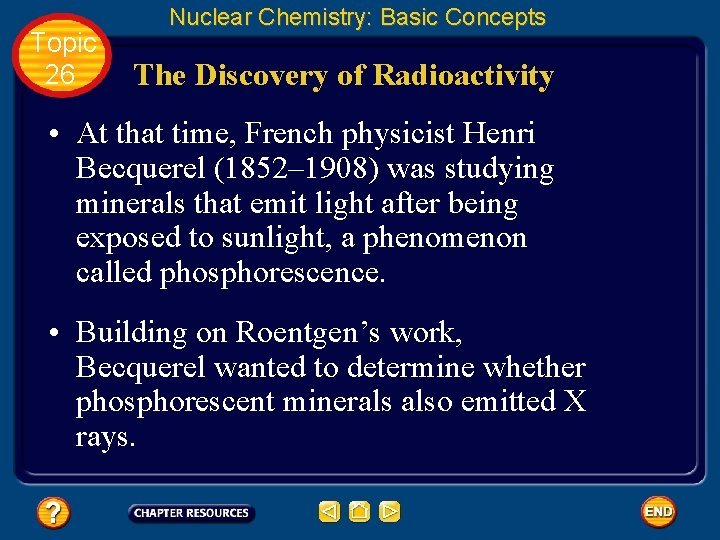Topic 26 Nuclear Chemistry: Basic Concepts The Discovery of Radioactivity • At that time, French physicist Henri Becquerel (1852– 1908) was studying minerals that emit light after being exposed to sunlight, a phenomenon called phosphorescence. • Building on Roentgen’s work, Becquerel wanted to determine whether phosphorescent minerals also emitted X rays.Topic 26 Nuclear Chemistry: Basic Concepts The Discovery of Radioactivity • Becquerel accidentally discovered that phosphorescent uranium salts—even when not exposed to light—produced spontaneous emissions that darkened photographic plates.Topic 26 Nuclear Chemistry: Basic Concepts The Discovery of Radioactivity • Marie Curie (1867– 1934) and her husband Pierre (1859 – 1906) took Becquerel’s mineral sample (called pitchblende) and isolated the components emitting the rays.Topic 26 Nuclear Chemistry: Basic Concepts The Discovery of Radioactivity • They concluded that the darkening of the photographic plates was due to rays emitted specifically from the uranium atoms present in the mineral sample. • Marie Curie named the process by which materials give off such rays radioactivity; the rays and particles emitted by a radioactive source are called radiation.Topic 26 Nuclear Chemistry: Basic Concepts Types of Radiation • As you may recall, isotopes are atoms of the same element that have different numbers of neutrons. • Isotopes of atoms with unstable nuclei are called radioisotopes.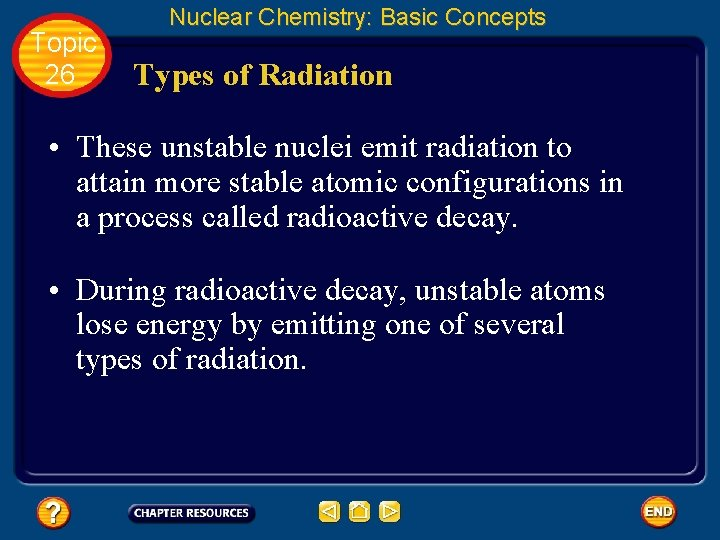Topic 26 Nuclear Chemistry: Basic Concepts Types of Radiation • These unstable nuclei emit radiation to attain more stable atomic configurations in a process called radioactive decay. • During radioactive decay, unstable atoms lose energy by emitting one of several types of radiation.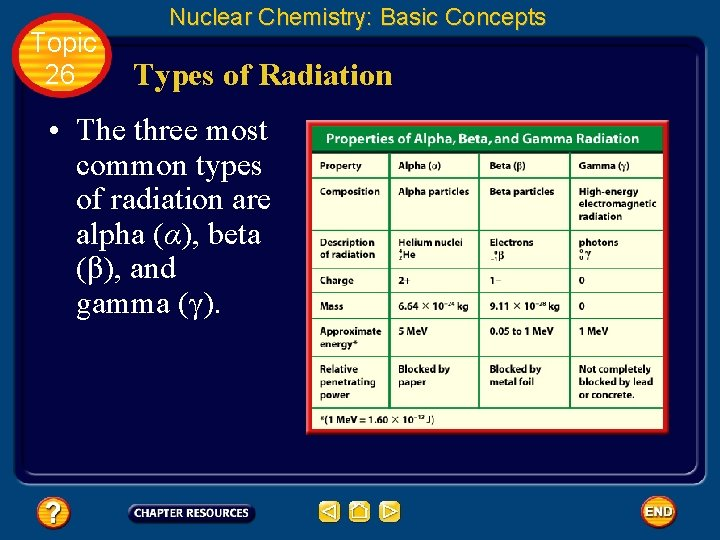Topic 26 Nuclear Chemistry: Basic Concepts Types of Radiation • The three most common types of radiation are alpha (α), beta (β), and gamma (γ).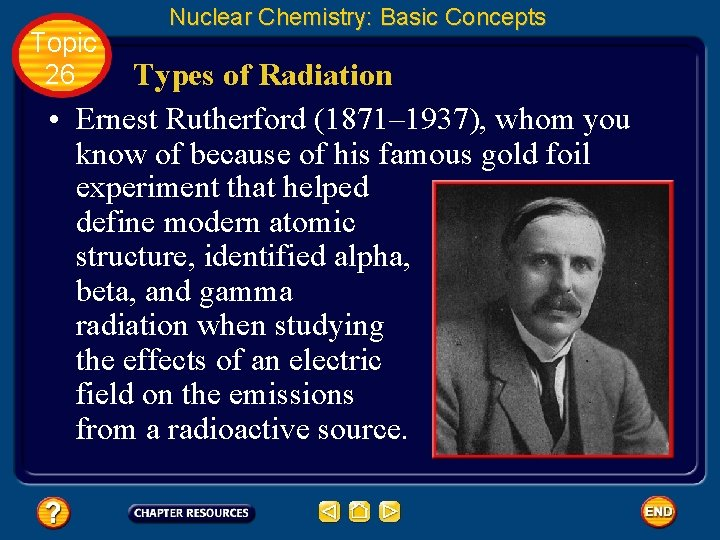Topic 26 Nuclear Chemistry: Basic Concepts Types of Radiation • Ernest Rutherford (1871– 1937), whom you know of because of his famous gold foil experiment that helped define modern atomic structure, identified alpha, beta, and gamma radiation when studying the effects of an electric field on the emissions from a radioactive source.Topic 26 Nuclear Chemistry: Basic Concepts Types of Radiation • The effect of an electric field on three types of radiation is shown. • Positively charged alpha particles are deflected toward the negatively charged plate.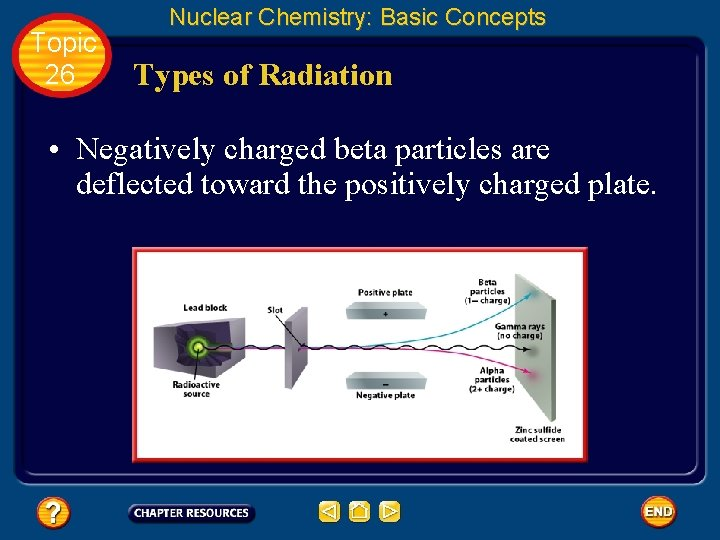Topic 26 Nuclear Chemistry: Basic Concepts Types of Radiation • Negatively charged beta particles are deflected toward the positively charged plate.Topic 26 Nuclear Chemistry: Basic Concepts Types of Radiation • Beta particles undergo greater deflection because they have considerably less mass than alpha particles.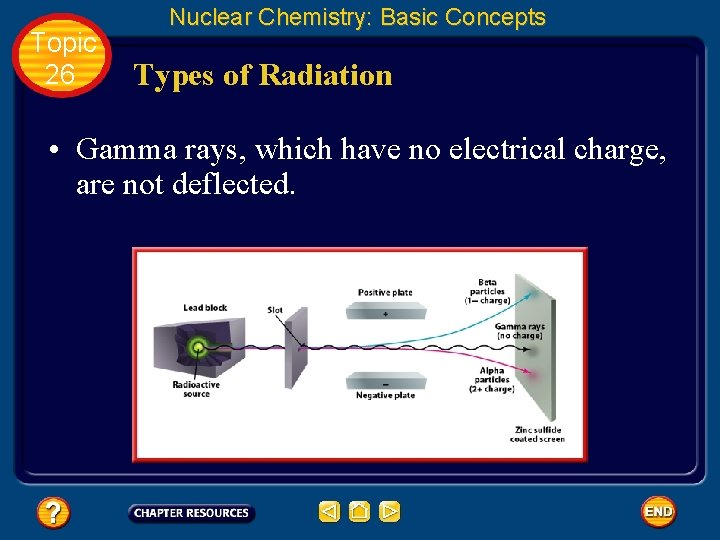Topic 26 Nuclear Chemistry: Basic Concepts Types of Radiation • Gamma rays, which have no electrical charge, are not deflected.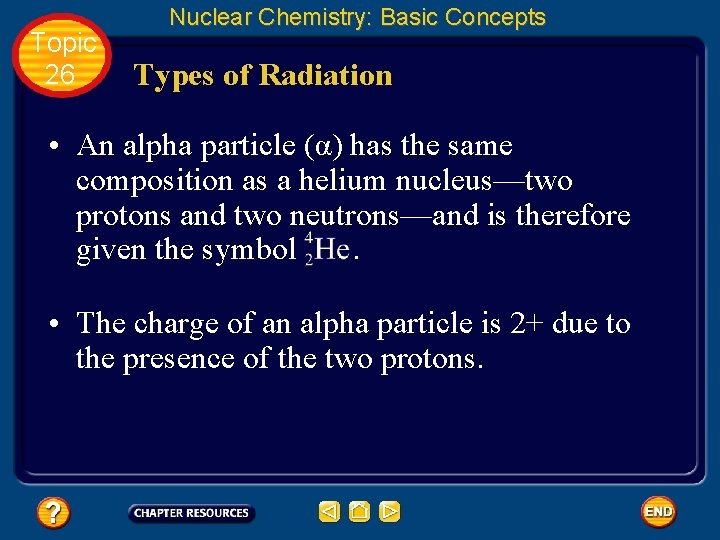Topic 26 Nuclear Chemistry: Basic Concepts Types of Radiation • An alpha particle (α) has the same composition as a helium nucleus—two protons and two neutrons—and is therefore given the symbol . • The charge of an alpha particle is 2+ due to the presence of the two protons.Topic 26 Nuclear Chemistry: Basic Concepts Types of Radiation • Alpha radiation consists of a stream of alpha particles. • Radium-226, an atom whose nucleus contains 88 protons and 138 neutrons, undergoes alpha decay by emitting an alpha particle.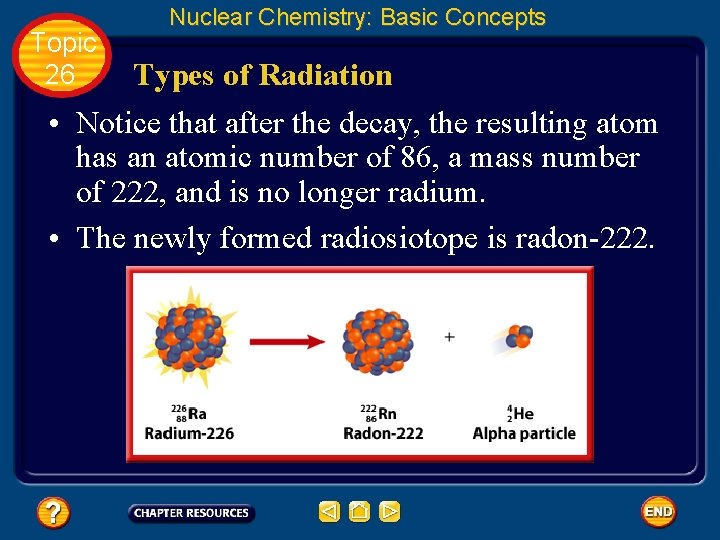Topic 26 Nuclear Chemistry: Basic Concepts Types of Radiation • Notice that after the decay, the resulting atom has an atomic number of 86, a mass number of 222, and is no longer radium. • The newly formed radiosiotope is radon-222.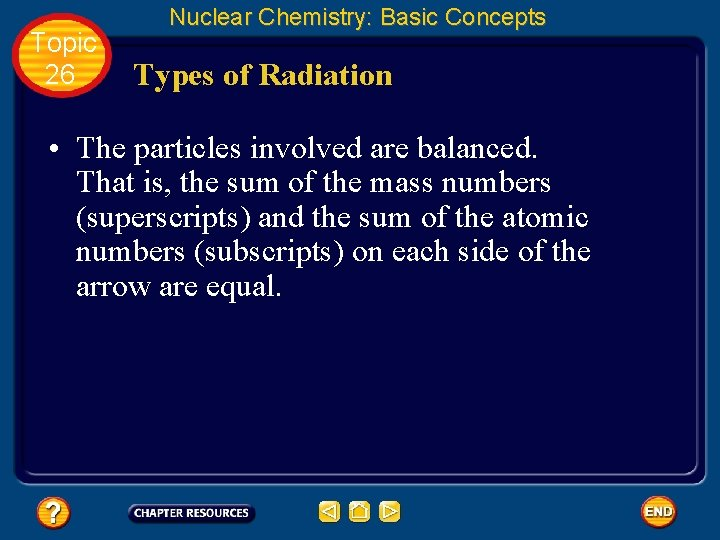Topic 26 Nuclear Chemistry: Basic Concepts Types of Radiation • The particles involved are balanced. That is, the sum of the mass numbers (superscripts) and the sum of the atomic numbers (subscripts) on each side of the arrow are equal.Topic 26 Nuclear Chemistry: Basic Concepts Types of Radiation • Because of their mass and charge, alpha particles are relatively slow-moving compared with other types of radiation. • Thus, alpha particles are not very penetrating—a single sheet of paper stops alpha particles.Topic 26 Nuclear Chemistry: Basic Concepts Types of Radiation • A beta particle is a very-fast moving electron that has been emitted from a neutron of an unstable nucleus. • Beta particles are represented by the symbol. The zero superscript indicates the insignificant mass of an electron in comparison with the mass of a nucleus.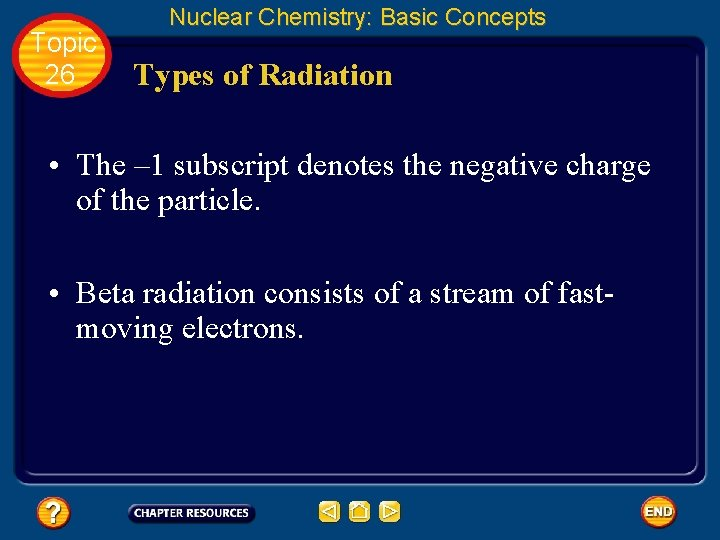Topic 26 Nuclear Chemistry: Basic Concepts Types of Radiation • The – 1 subscript denotes the negative charge of the particle. • Beta radiation consists of a stream of fastmoving electrons.Topic 26 Nuclear Chemistry: Basic Concepts Types of Radiation • An example of the beta decay process is the decay of iodine-131 into xenon-131 by betaparticle emission.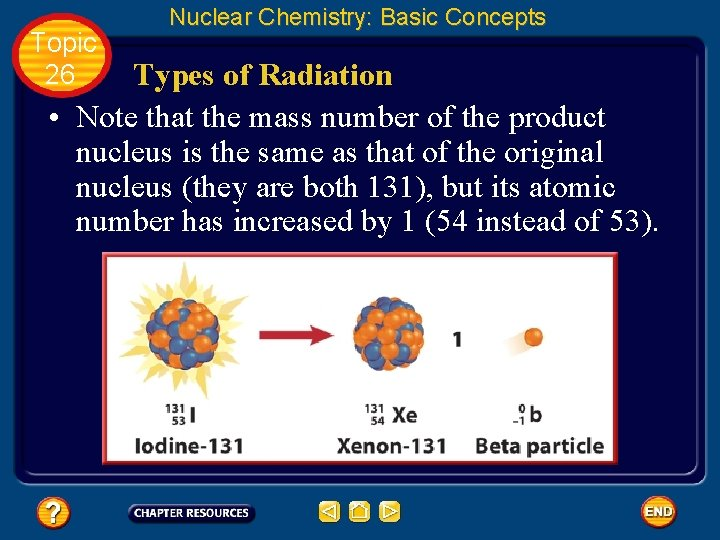Topic 26 Nuclear Chemistry: Basic Concepts Types of Radiation • Note that the mass number of the product nucleus is the same as that of the original nucleus (they are both 131), but its atomic number has increased by 1 (54 instead of 53).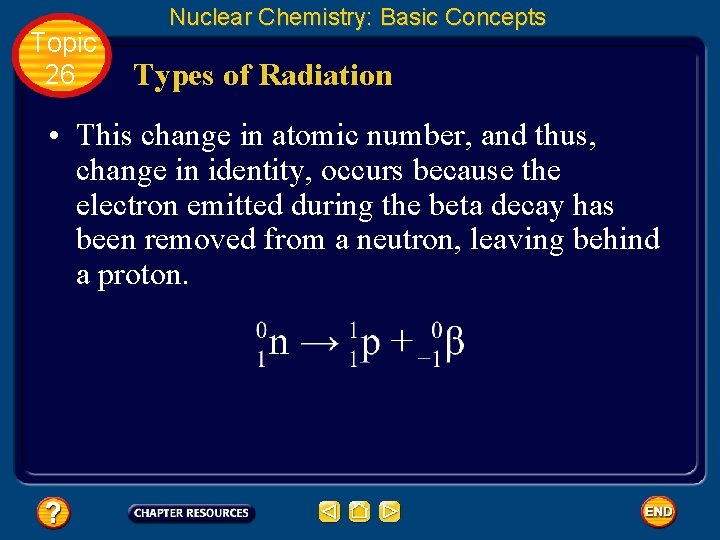Topic 26 Nuclear Chemistry: Basic Concepts Types of Radiation • This change in atomic number, and thus, change in identity, occurs because the electron emitted during the beta decay has been removed from a neutron, leaving behind a proton.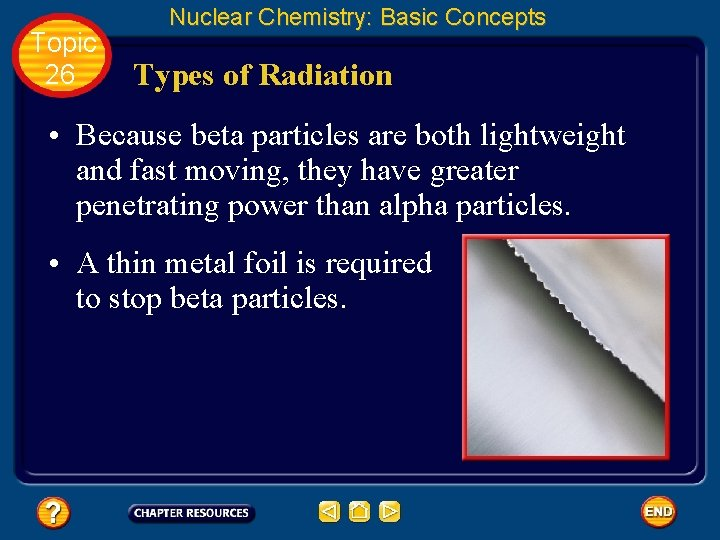Topic 26 Nuclear Chemistry: Basic Concepts Types of Radiation • Because beta particles are both lightweight and fast moving, they have greater penetrating power than alpha particles. • A thin metal foil is required to stop beta particles.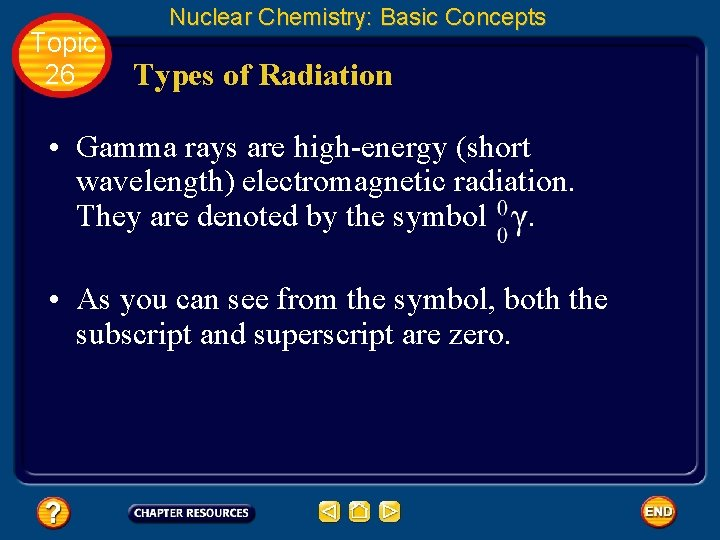Topic 26 Nuclear Chemistry: Basic Concepts Types of Radiation • Gamma rays are high-energy (short wavelength) electromagnetic radiation. They are denoted by the symbol . • As you can see from the symbol, both the subscript and superscript are zero.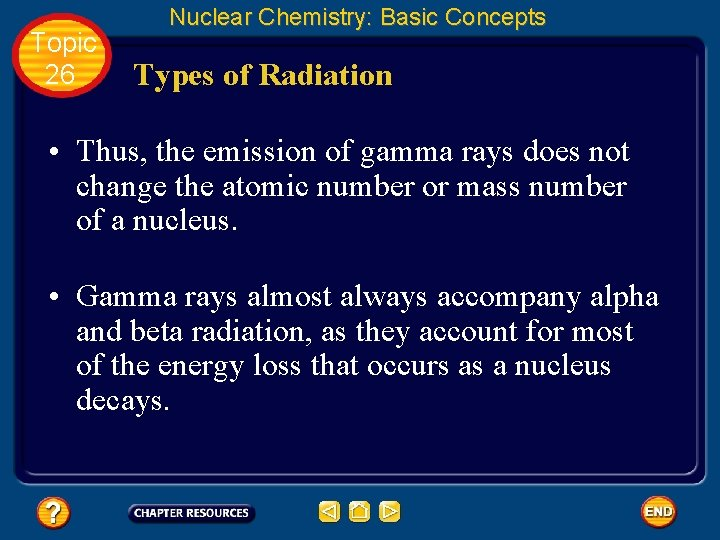Topic 26 Nuclear Chemistry: Basic Concepts Types of Radiation • Thus, the emission of gamma rays does not change the atomic number or mass number of a nucleus. • Gamma rays almost always accompany alpha and beta radiation, as they account for most of the energy loss that occurs as a nucleus decays.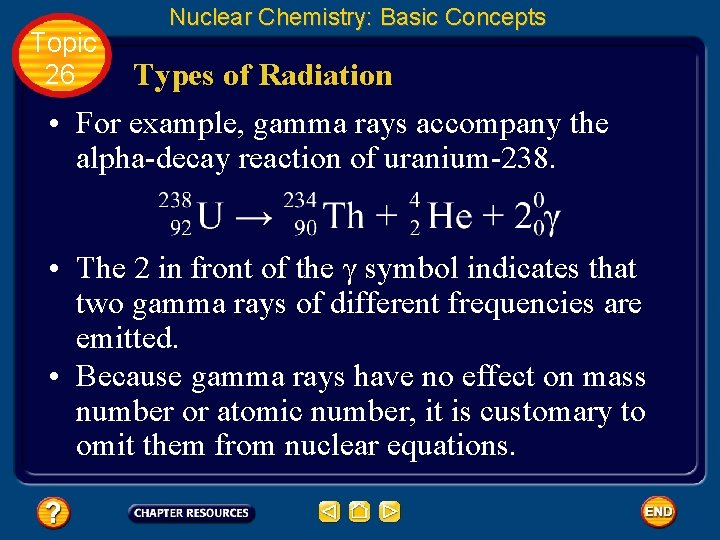Topic 26 Nuclear Chemistry: Basic Concepts Types of Radiation • For example, gamma rays accompany the alpha-decay reaction of uranium-238. • The 2 in front of the γ symbol indicates that two gamma rays of different frequencies are emitted. • Because gamma rays have no effect on mass number or atomic number, it is customary to omit them from nuclear equations.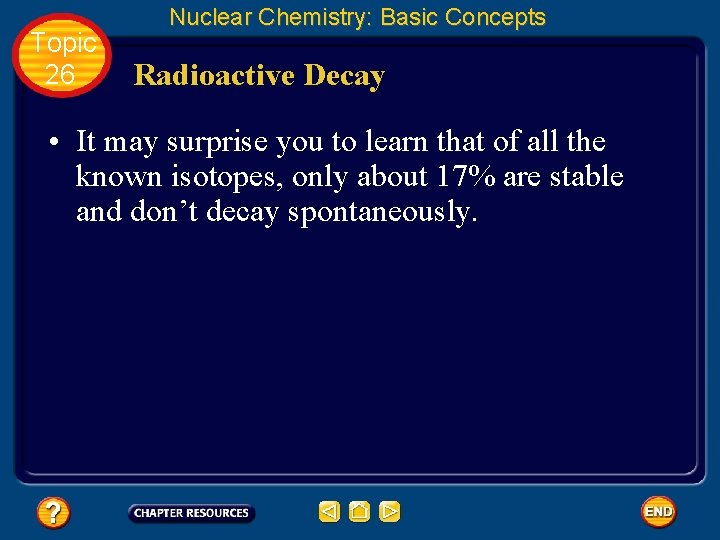Topic 26 Nuclear Chemistry: Basic Concepts Radioactive Decay • It may surprise you to learn that of all the known isotopes, only about 17% are stable and don’t decay spontaneously.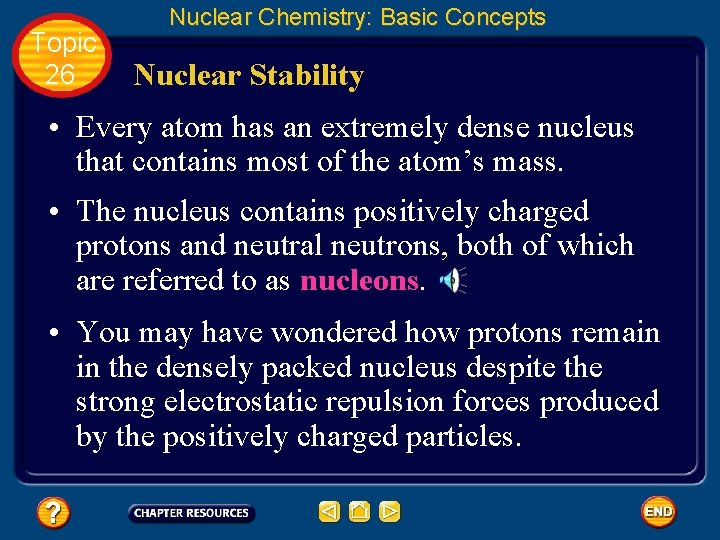Topic 26 Nuclear Chemistry: Basic Concepts Nuclear Stability • Every atom has an extremely dense nucleus that contains most of the atom’s mass. • The nucleus contains positively charged protons and neutral neutrons, both of which are referred to as nucleons. • You may have wondered how protons remain in the densely packed nucleus despite the strong electrostatic repulsion forces produced by the positively charged particles.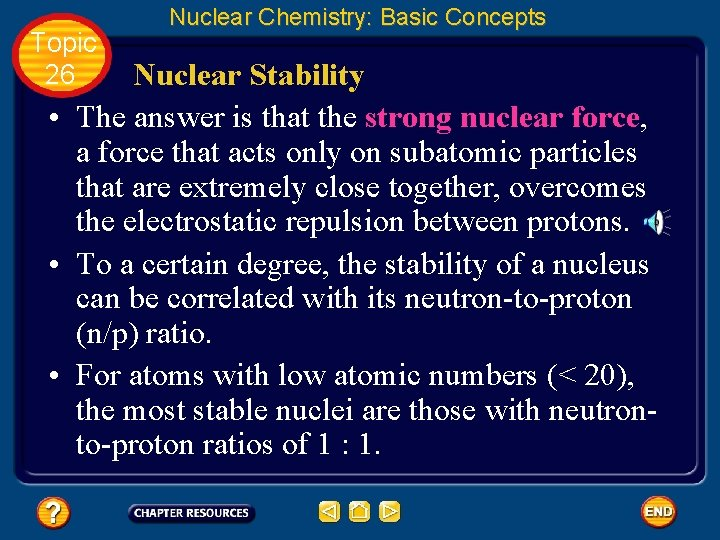Topic 26 Nuclear Chemistry: Basic Concepts Nuclear Stability • The answer is that the strong nuclear force, a force that acts only on subatomic particles that are extremely close together, overcomes the electrostatic repulsion between protons. • To a certain degree, the stability of a nucleus can be correlated with its neutron-to-proton (n/p) ratio. • For atoms with low atomic numbers (< 20), the most stable nuclei are those with neutronto-proton ratios of 1 : 1.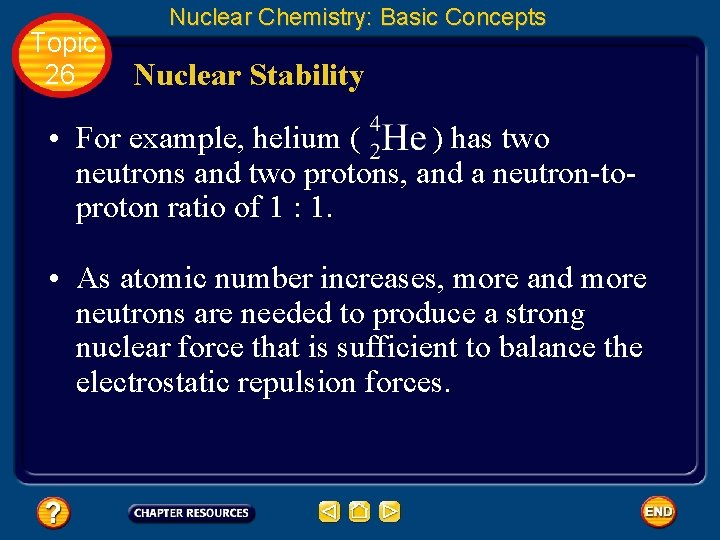Topic 26 Nuclear Chemistry: Basic Concepts Nuclear Stability • For example, helium ( ) has two neutrons and two protons, and a neutron-toproton ratio of 1 : 1. • As atomic number increases, more and more neutrons are needed to produce a strong nuclear force that is sufficient to balance the electrostatic repulsion forces.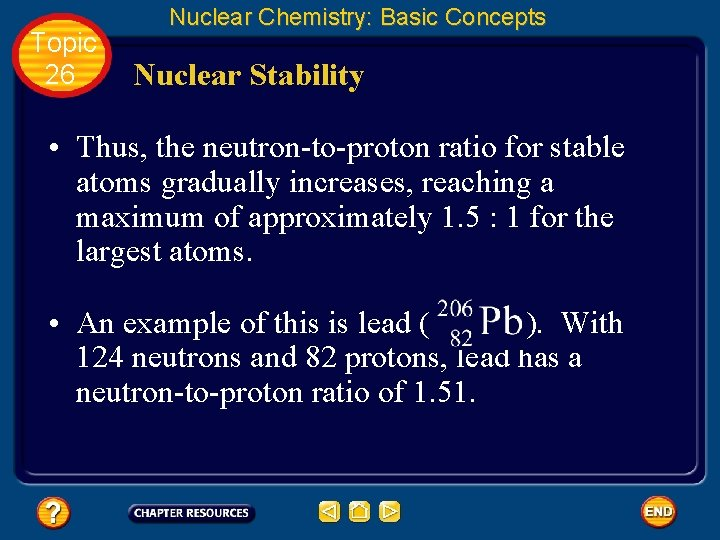Topic 26 Nuclear Chemistry: Basic Concepts Nuclear Stability • Thus, the neutron-to-proton ratio for stable atoms gradually increases, reaching a maximum of approximately 1. 5 : 1 for the largest atoms. • An example of this is lead ( ). With 124 neutrons and 82 protons, lead has a neutron-to-proton ratio of 1. 51.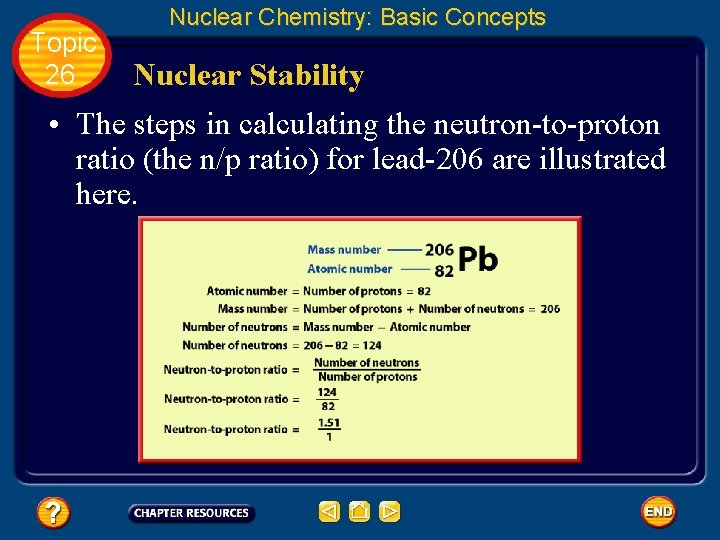Topic 26 Nuclear Chemistry: Basic Concepts Nuclear Stability • The steps in calculating the neutron-to-proton ratio (the n/p ratio) for lead-206 are illustrated here.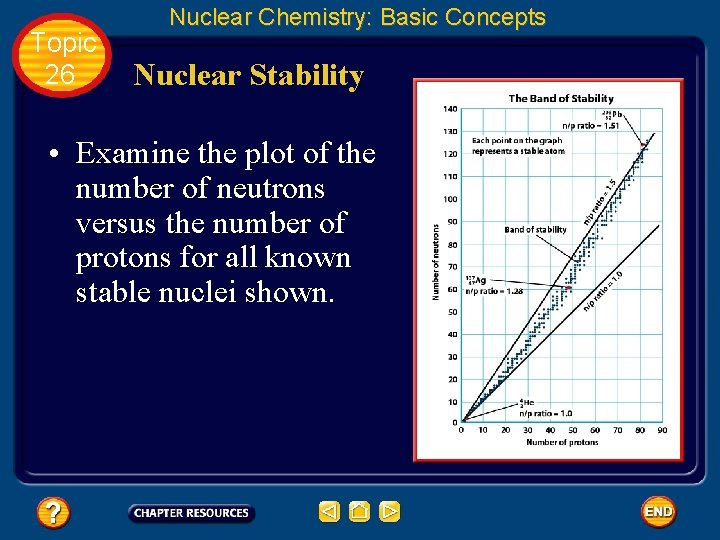Topic 26 Nuclear Chemistry: Basic Concepts Nuclear Stability • Examine the plot of the number of neutrons versus the number of protons for all known stable nuclei shown.Topic 26 Nuclear Chemistry: Basic Concepts Nuclear Stability • As you can see, the slope of the plot indicates that the number of neutrons required for a stable nucleus increases as the number of protons increases. • This correlates with the increase in the neutron -to-proton ratio of stable nuclei with increasing atomic number. • The area on the graph within which all stable nuclei are found is known as the band of stability.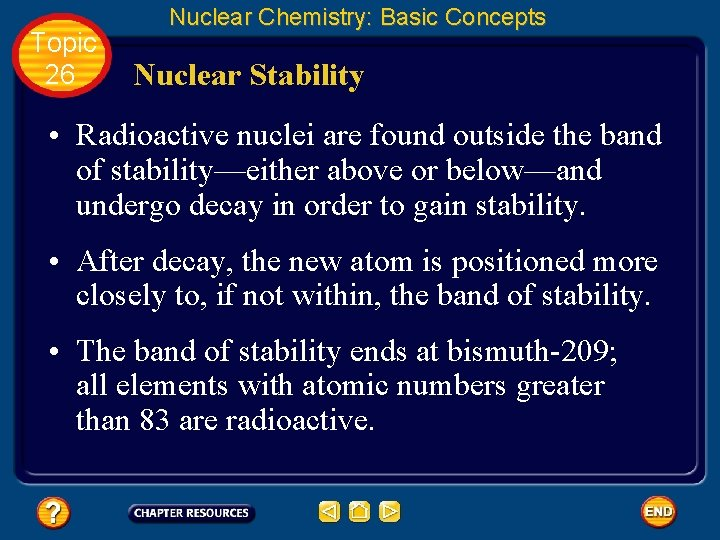Topic 26 Nuclear Chemistry: Basic Concepts Nuclear Stability • Radioactive nuclei are found outside the band of stability—either above or below—and undergo decay in order to gain stability. • After decay, the new atom is positioned more closely to, if not within, the band of stability. • The band of stability ends at bismuth-209; all elements with atomic numbers greater than 83 are radioactive.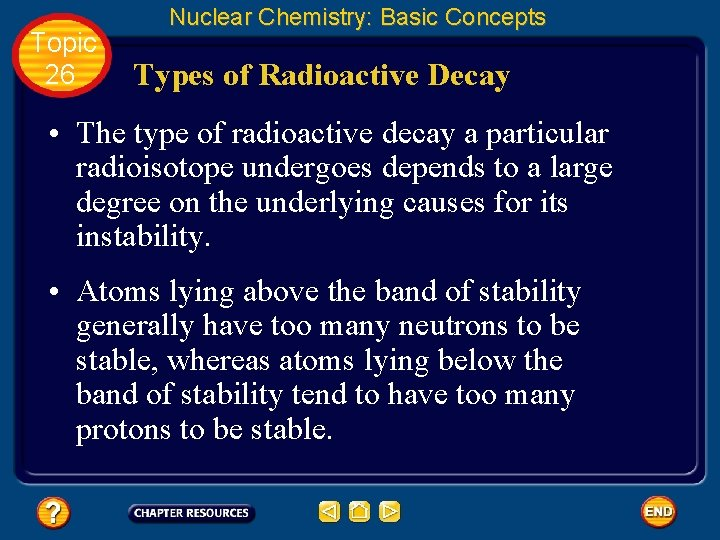Topic 26 Nuclear Chemistry: Basic Concepts Types of Radioactive Decay • The type of radioactive decay a particular radioisotope undergoes depends to a large degree on the underlying causes for its instability. • Atoms lying above the band of stability generally have too many neutrons to be stable, whereas atoms lying below the band of stability tend to have too many protons to be stable.Topic 26 Nuclear Chemistry: Basic Concepts Beta Decay • A radioisotope that lies above the band of stability is unstable because it has too many neutrons relative to its number of protons. • For example, unstable has a neutron-toproton ratio of 1. 33 : 1, whereas stable elements of similar mass, such as and , have neutron-to-proton ratios of approximately 1 : 1.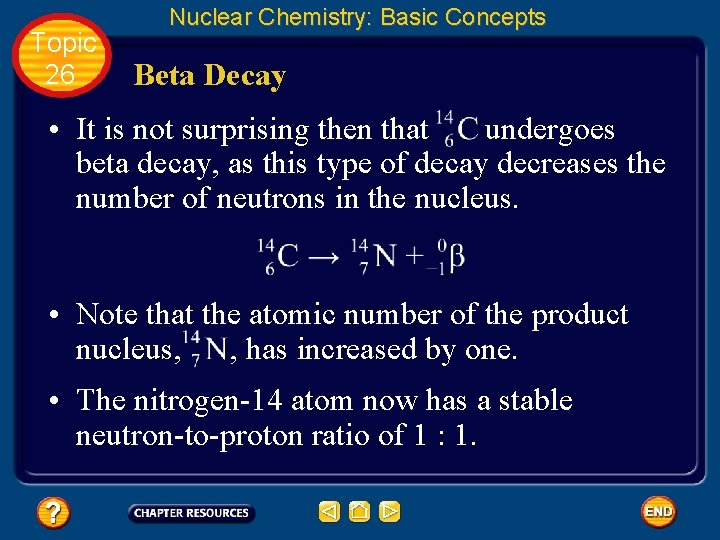Topic 26 Nuclear Chemistry: Basic Concepts Beta Decay • It is not surprising then that undergoes beta decay, as this type of decay decreases the number of neutrons in the nucleus. • Note that the atomic number of the product nucleus, , has increased by one. • The nitrogen-14 atom now has a stable neutron-to-proton ratio of 1 : 1.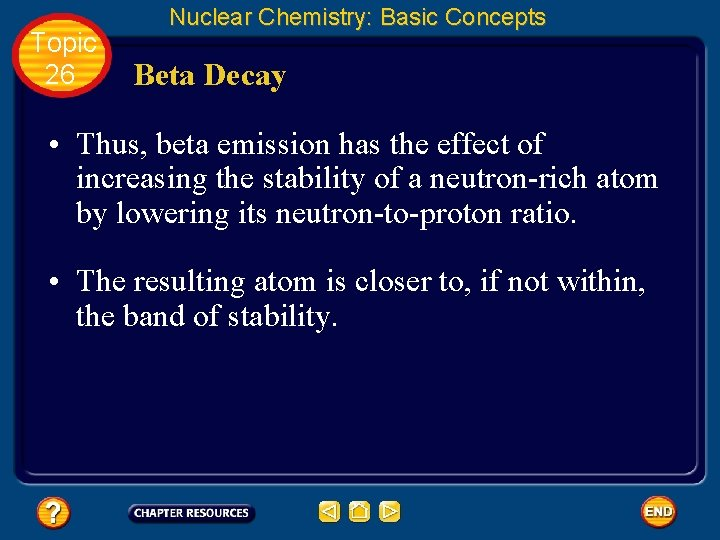Topic 26 Nuclear Chemistry: Basic Concepts Beta Decay • Thus, beta emission has the effect of increasing the stability of a neutron-rich atom by lowering its neutron-to-proton ratio. • The resulting atom is closer to, if not within, the band of stability.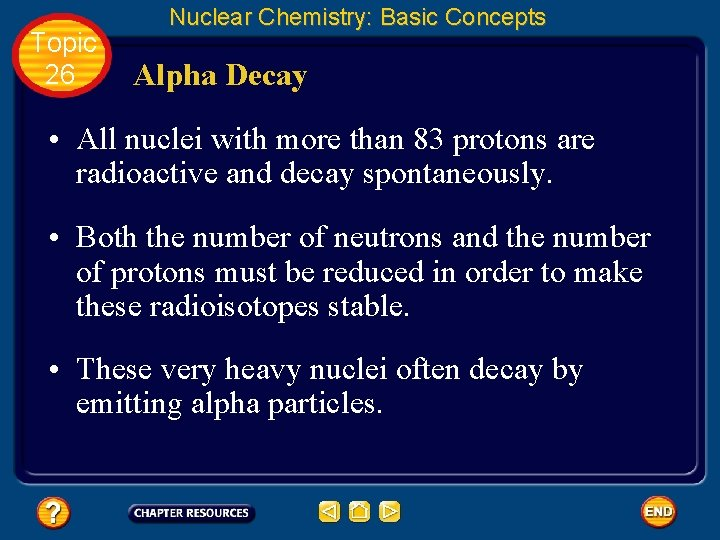Topic 26 Nuclear Chemistry: Basic Concepts Alpha Decay • All nuclei with more than 83 protons are radioactive and decay spontaneously. • Both the number of neutrons and the number of protons must be reduced in order to make these radioisotopes stable. • These very heavy nuclei often decay by emitting alpha particles.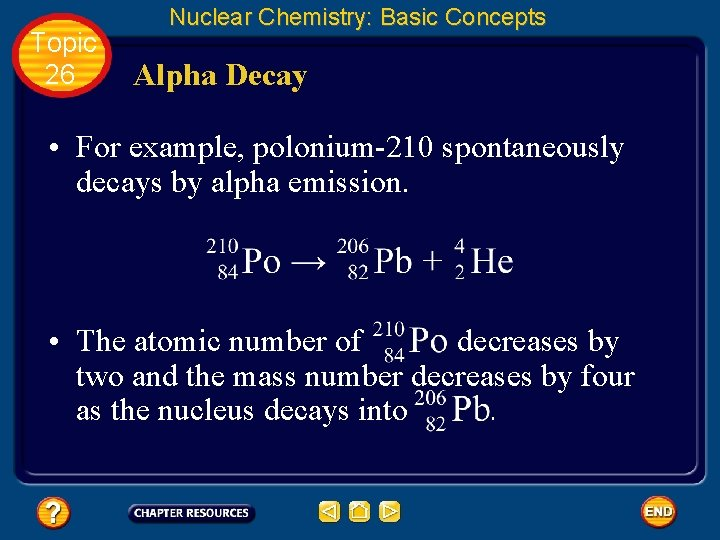Topic 26 Nuclear Chemistry: Basic Concepts Alpha Decay • For example, polonium-210 spontaneously decays by alpha emission. • The atomic number of decreases by two and the mass number decreases by four as the nucleus decays into .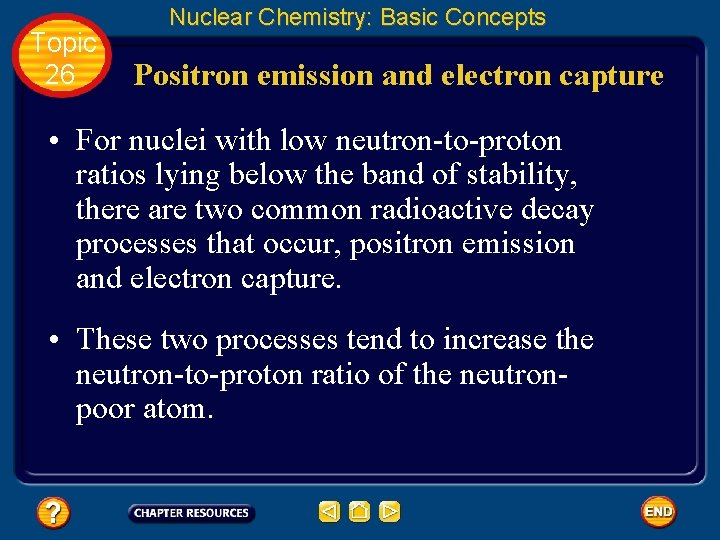Topic 26 Nuclear Chemistry: Basic Concepts Positron emission and electron capture • For nuclei with low neutron-to-proton ratios lying below the band of stability, there are two common radioactive decay processes that occur, positron emission and electron capture. • These two processes tend to increase the neutron-to-proton ratio of the neutronpoor atom.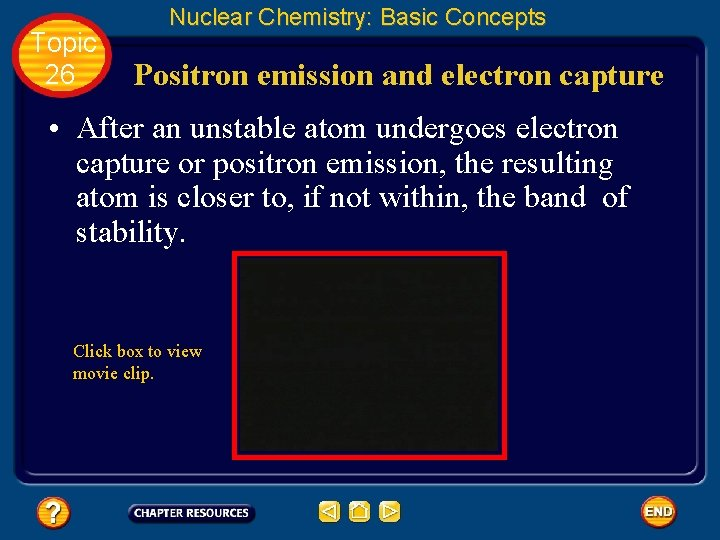Topic 26 Nuclear Chemistry: Basic Concepts Positron emission and electron capture • After an unstable atom undergoes electron capture or positron emission, the resulting atom is closer to, if not within, the band of stability. Click box to view movie clip.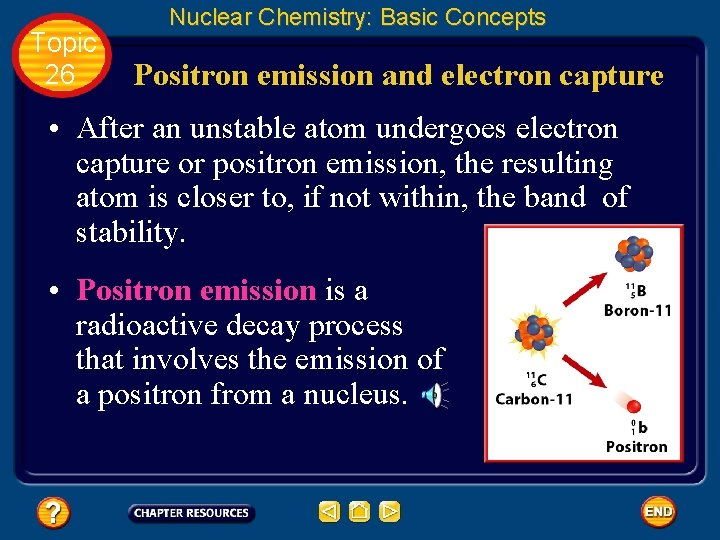Topic 26 Nuclear Chemistry: Basic Concepts Positron emission and electron capture • After an unstable atom undergoes electron capture or positron emission, the resulting atom is closer to, if not within, the band of stability. • Positron emission is a radioactive decay process that involves the emission of a positron from a nucleus.Topic 26 Nuclear Chemistry: Basic Concepts Positron emission and electron capture • A positron is a particle with the same mass as an electron but opposite charge, thus it is represented by the symbol . • During positron emission, a proton in the nucleus is converted into a neutron and a positron, and then the positron is emitted.Topic 26 Nuclear Chemistry: Basic Concepts Positron emission and electron capture • Electron capture is the other common radioactive decay process that decreases the number of protons in unstable nuclei lying below the band of stability.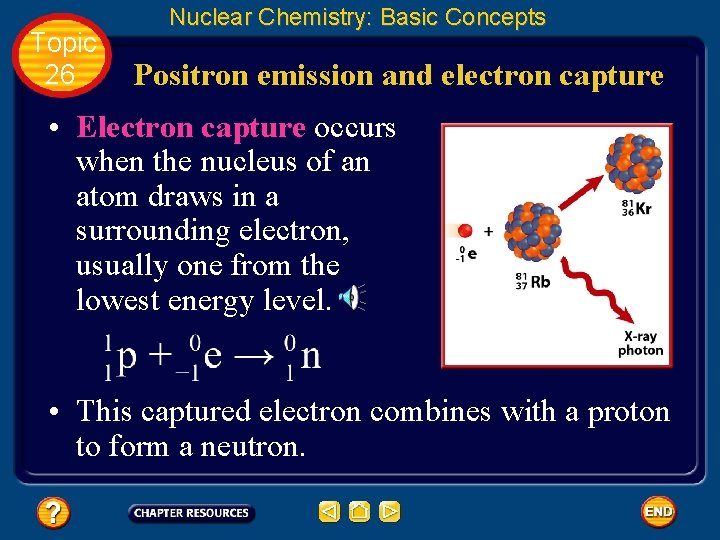Topic 26 Nuclear Chemistry: Basic Concepts Positron emission and electron capture • Electron capture occurs when the nucleus of an atom draws in a surrounding electron, usually one from the lowest energy level. • This captured electron combines with a proton to form a neutron.Topic 26 Nuclear Chemistry: Basic Concepts Balancing a Nuclear Equation • Write a balanced nuclear equation for the alpha decay of thorium-230 ( ). • You are given that a thorium atom undergoes alpha decay and forms an unknown product. • Thorium-230 is the initial reactant, while the alpha particle is one of the products of the reaction. The reaction is summarized below.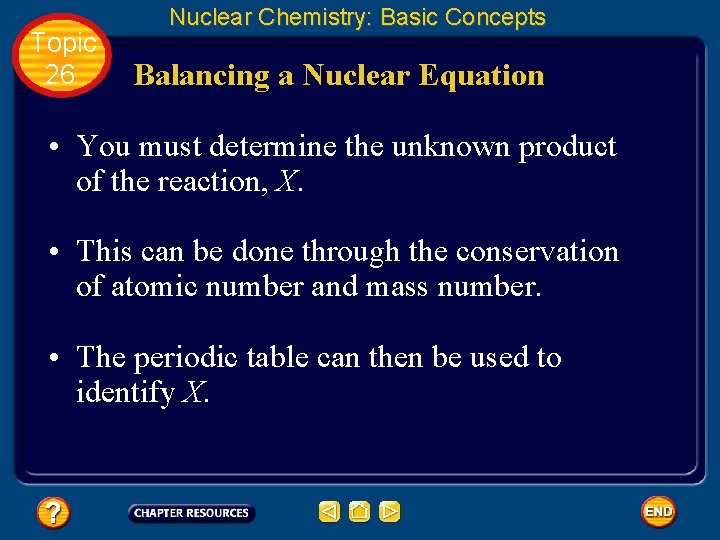Topic 26 Nuclear Chemistry: Basic Concepts Balancing a Nuclear Equation • You must determine the unknown product of the reaction, X. • This can be done through the conservation of atomic number and mass number. • The periodic table can then be used to identify X.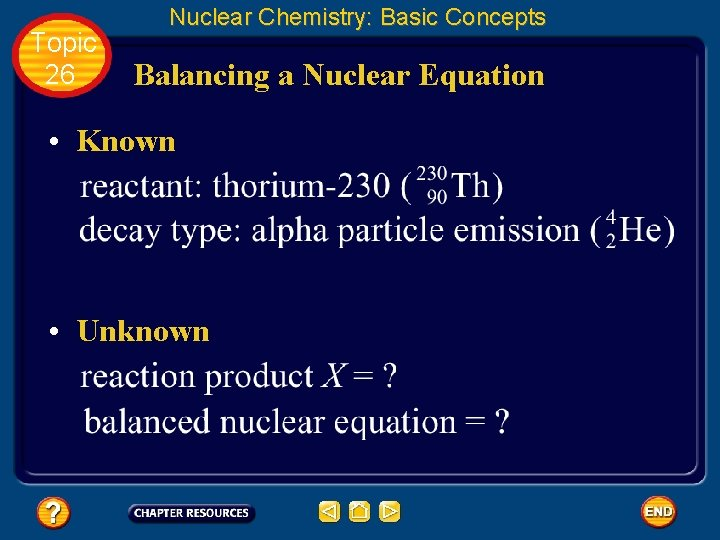Topic 26 Nuclear Chemistry: Basic Concepts Balancing a Nuclear Equation • Known • UnknownTopic 26 Nuclear Chemistry: Basic Concepts Balancing a Nuclear Equation • Using each particle’s mass number, make sure mass number is conserved on each side of the reaction arrow. • Thus, the mass number of X is 226. • Using each particle’s atomic number, make sure atomic number is conserved on each side of the reaction arrow.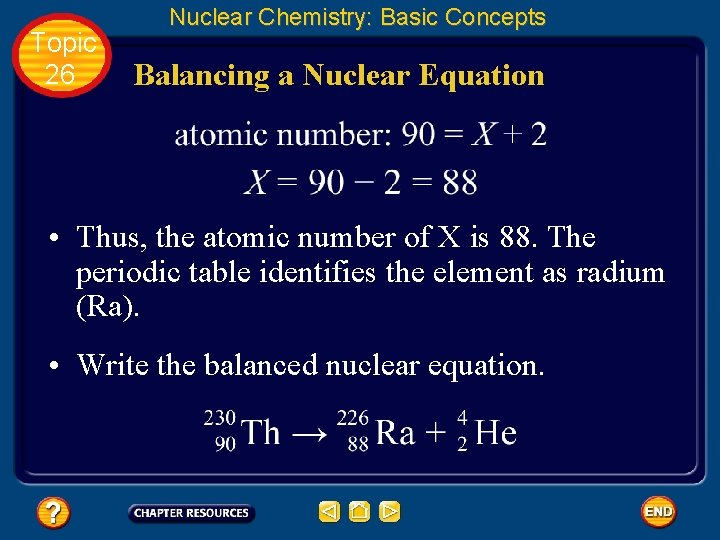Topic 26 Nuclear Chemistry: Basic Concepts Balancing a Nuclear Equation • Thus, the atomic number of X is 88. The periodic table identifies the element as radium (Ra). • Write the balanced nuclear equation.Topic 26 Basic Assessment Questions Question 1 What element is formed when polonium-214 ( ) radioisotope undergoes alpha decay? Give the atomic number and mass number of the element.Topic 26 Basic Assessment Questions Answer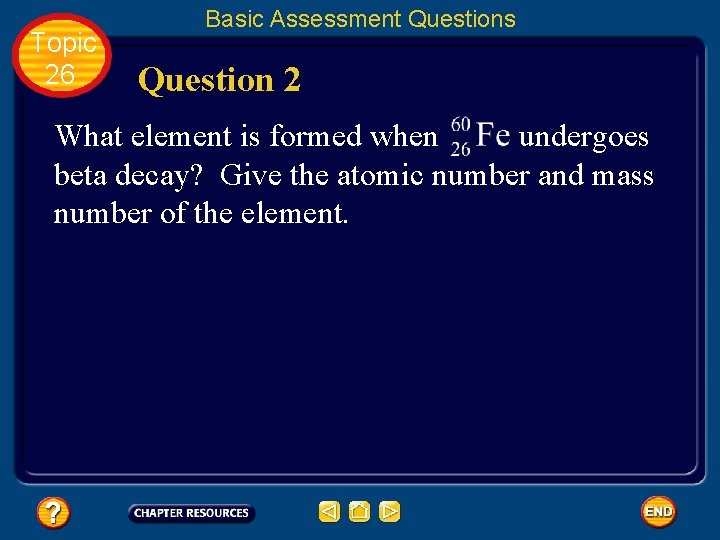Topic 26 Basic Assessment Questions Question 2 What element is formed when undergoes beta decay? Give the atomic number and mass number of the element.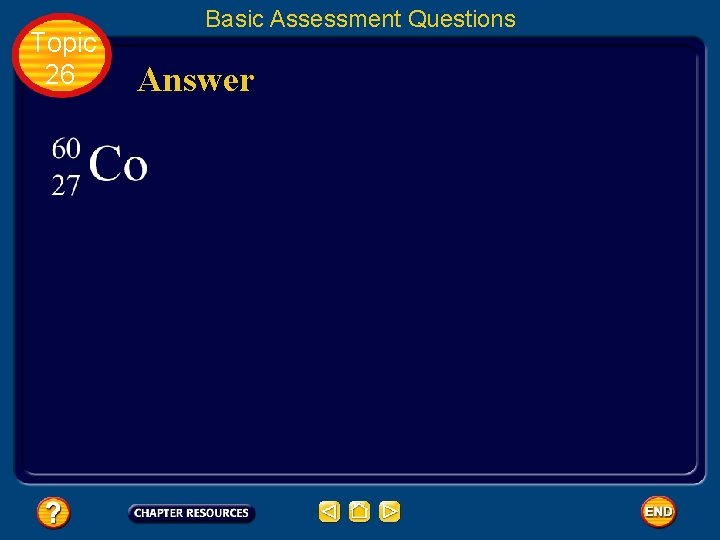Topic 26 Basic Assessment Questions Answer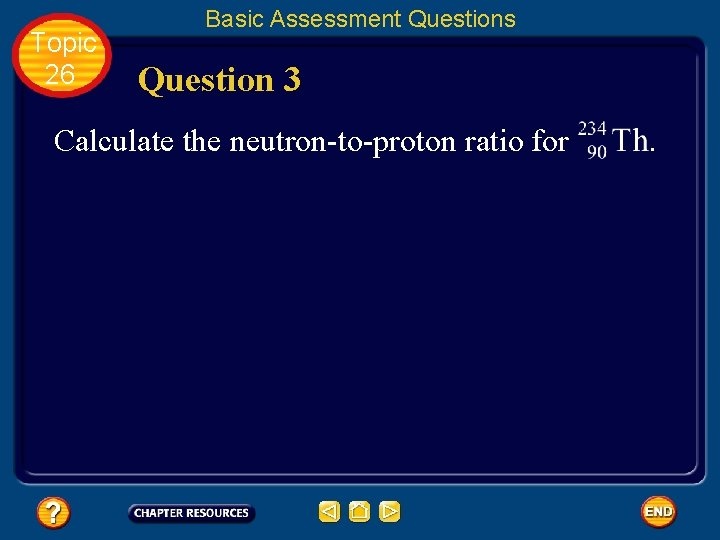Topic 26 Basic Assessment Questions Question 3 Calculate the neutron-to-proton ratio for .Topic 26 1. 6 : 1 Basic Assessment Questions Answer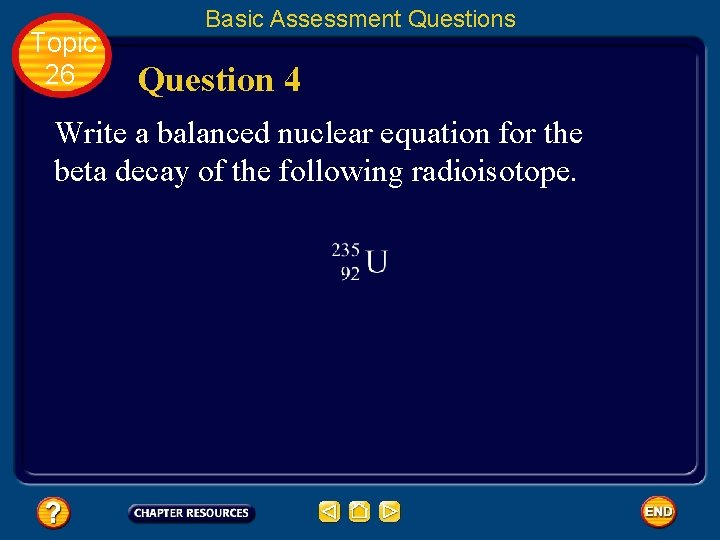Topic 26 Basic Assessment Questions Question 4 Write a balanced nuclear equation for the beta decay of the following radioisotope.Topic 26 Basic Assessment Questions AnswerTopic 26 Nuclear Chemistry: Additional Concepts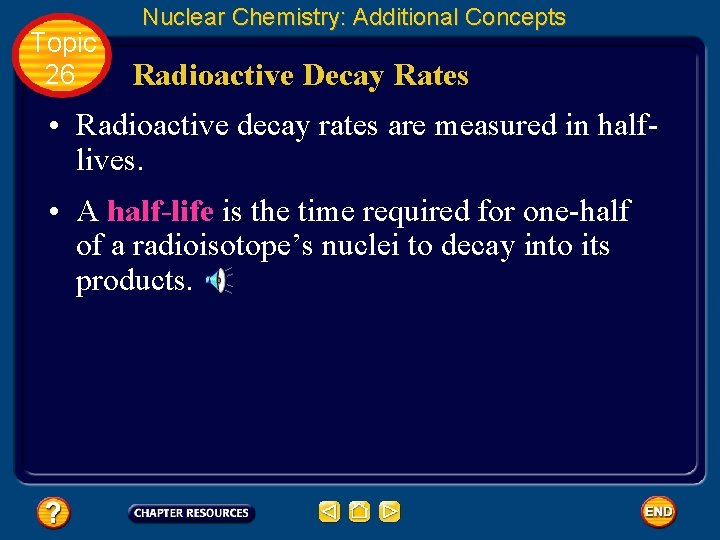Topic 26 Nuclear Chemistry: Additional Concepts Radioactive Decay Rates • Radioactive decay rates are measured in halflives. • A half-life is the time required for one-half of a radioisotope’s nuclei to decay into its products.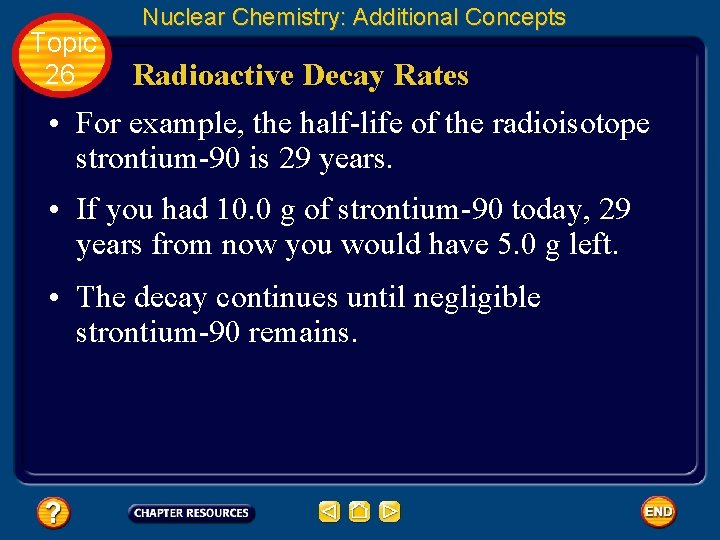Topic 26 Nuclear Chemistry: Additional Concepts Radioactive Decay Rates • For example, the half-life of the radioisotope strontium-90 is 29 years. • If you had 10. 0 g of strontium-90 today, 29 years from now you would have 5. 0 g left. • The decay continues until negligible strontium-90 remains.Topic 26 Nuclear Chemistry: Additional Concepts Radioactive Decay Rates • The graph shows the percent of a stontium-90 sample remaining over a period of four halflives. • With the passing of each half-life, half of the strontium-90 sample decays.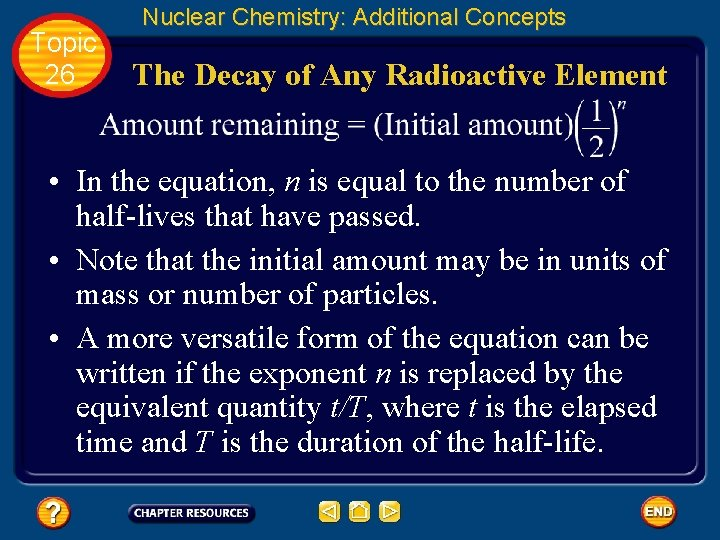Topic 26 Nuclear Chemistry: Additional Concepts The Decay of Any Radioactive Element • In the equation, n is equal to the number of half-lives that have passed. • Note that the initial amount may be in units of mass or number of particles. • A more versatile form of the equation can be written if the exponent n is replaced by the equivalent quantity t/T, where t is the elapsed time and T is the duration of the half-life.Topic 26 Nuclear Chemistry: Additional Concepts The Decay of Any Radioactive Element • Note that both t and T must have the same units of time. • This type of expression is known as an exponential decay function. • Each radioisotope has its own characteristic half-life.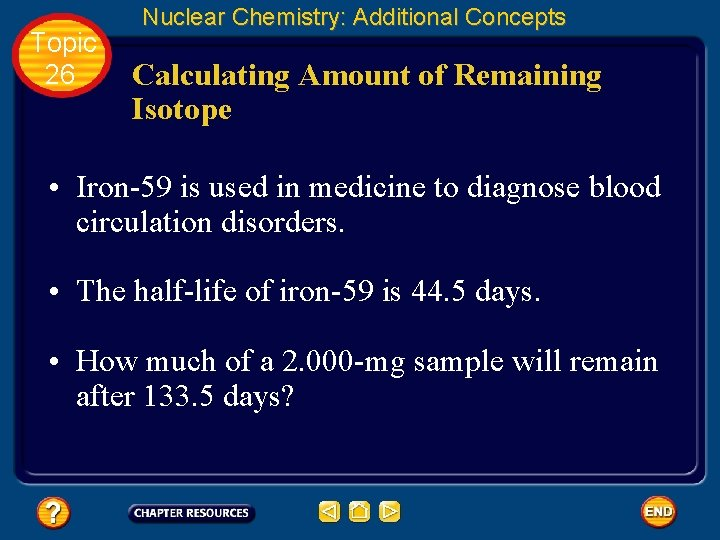Topic 26 Nuclear Chemistry: Additional Concepts Calculating Amount of Remaining Isotope • Iron-59 is used in medicine to diagnose blood circulation disorders. • The half-life of iron-59 is 44. 5 days. • How much of a 2. 000 -mg sample will remain after 133. 5 days?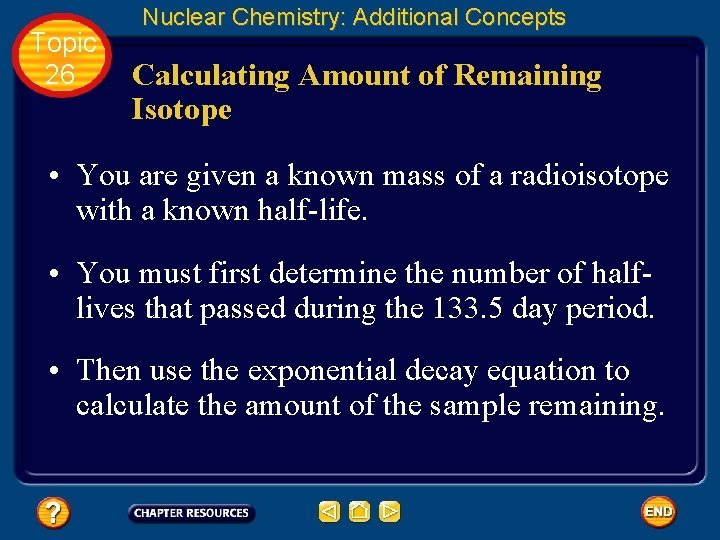Topic 26 Nuclear Chemistry: Additional Concepts Calculating Amount of Remaining Isotope • You are given a known mass of a radioisotope with a known half-life. • You must first determine the number of halflives that passed during the 133. 5 day period. • Then use the exponential decay equation to calculate the amount of the sample remaining.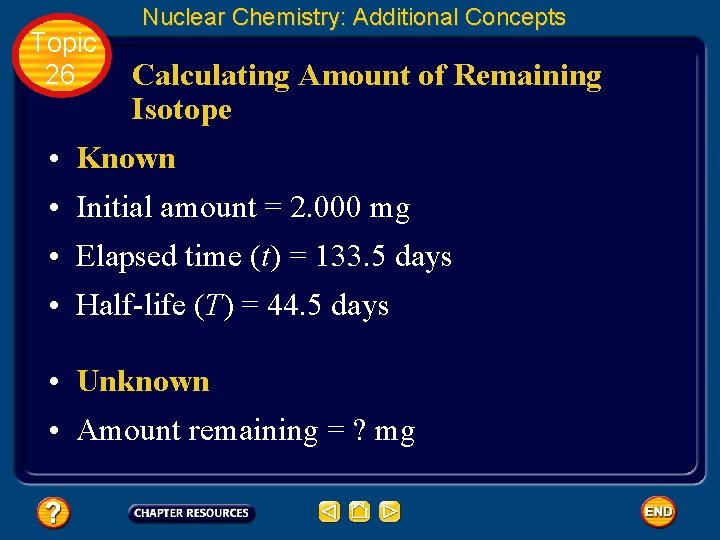Topic 26 Nuclear Chemistry: Additional Concepts Calculating Amount of Remaining Isotope • Known • Initial amount = 2. 000 mg • Elapsed time (t) = 133. 5 days • Half-life (T) = 44. 5 days • Unknown • Amount remaining = ? mgTopic 26 Nuclear Chemistry: Additional Concepts Calculating Amount of Remaining Isotope • Determine the number of half-lives passed during the 133. 5 days.Topic 26 Nuclear Chemistry: Additional Concepts Calculating Amount of Remaining Isotope • Substitute the values for n and initial mass into the exponential decay equation and solve.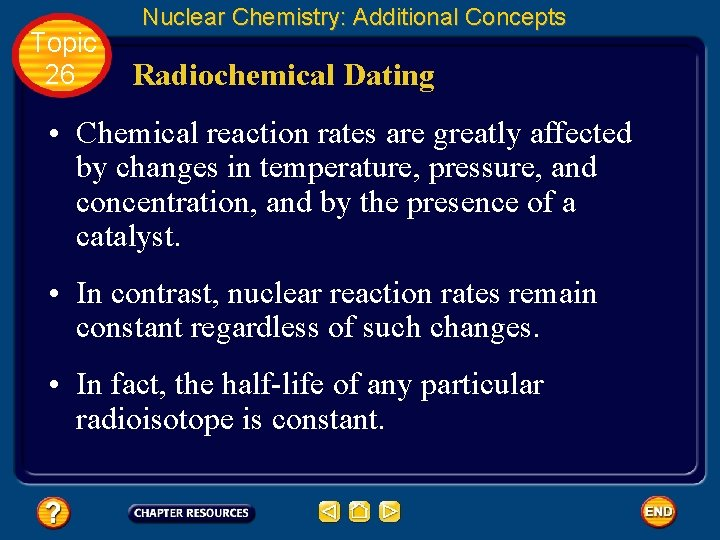Topic 26 Nuclear Chemistry: Additional Concepts Radiochemical Dating • Chemical reaction rates are greatly affected by changes in temperature, pressure, and concentration, and by the presence of a catalyst. • In contrast, nuclear reaction rates remain constant regardless of such changes. • In fact, the half-life of any particular radioisotope is constant.Topic 26 Nuclear Chemistry: Additional Concepts Radiochemical Dating • Because of this, radioisotopes can be used to determine the age of an object. • The process of determining the age of an object by measuring the amount of a certain radioisotope remaining in that object is called radiochemical dating. Click box to view movie clip.Topic 26 Nuclear Chemistry: Additional Concepts Nuclear fission • Heavy atoms (mass number > 60) tend to break into smaller atoms, thereby increasing their stability. • The splitting of a nucleus into fragments is called nuclear fission. • Nuclear fission releases a large amount of energy.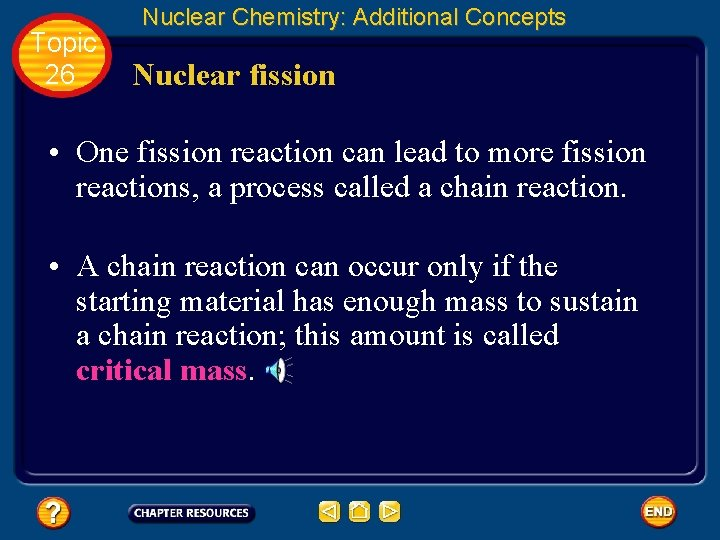Topic 26 Nuclear Chemistry: Additional Concepts Nuclear fission • One fission reaction can lead to more fission reactions, a process called a chain reaction. • A chain reaction can occur only if the starting material has enough mass to sustain a chain reaction; this amount is called critical mass.Topic 26 Nuclear Chemistry: Additional Concepts Nuclear reactors • Nuclear power plants use the process of nuclear fission to produce heat in nuclear reactors. • The heat is used to generate steam, which is then used to drive turbines that produce electricity.Topic 26 Nuclear Chemistry: Additional Concepts Nuclear reactors • Fissionable uranium(IV) oxide (UO 2) is commonly used as fuel in nuclear reactors. Click box to view movie clip.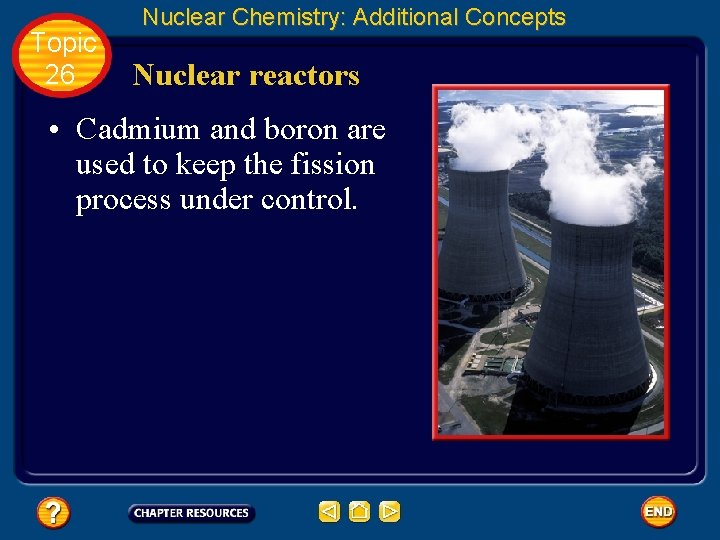Topic 26 Nuclear Chemistry: Additional Concepts Nuclear reactors • Cadmium and boron are used to keep the fission process under control.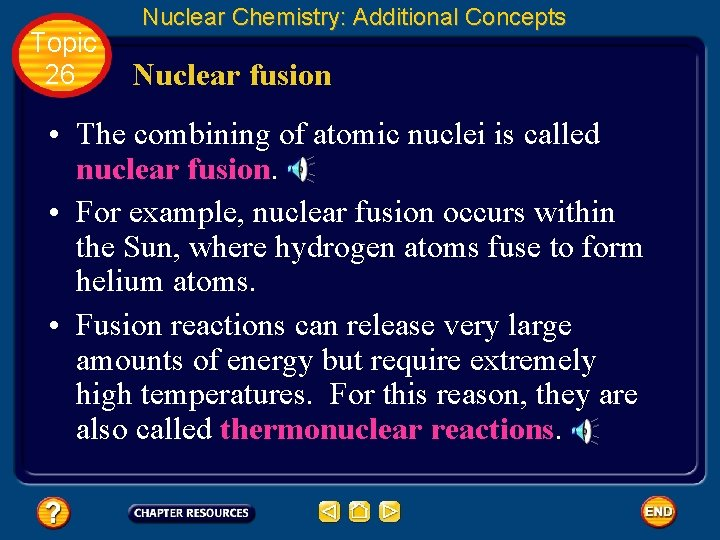Topic 26 Nuclear Chemistry: Additional Concepts Nuclear fusion • The combining of atomic nuclei is called nuclear fusion. • For example, nuclear fusion occurs within the Sun, where hydrogen atoms fuse to form helium atoms. • Fusion reactions can release very large amounts of energy but require extremely high temperatures. For this reason, they are also called thermonuclear reactions.Topic 26 Nuclear Chemistry: Additional Concepts Applications and Effects of Nuclear Reactions • Geiger counters, scintillation counters, and film badges are devices used to detect and measure radiation. Click box to view movie clip.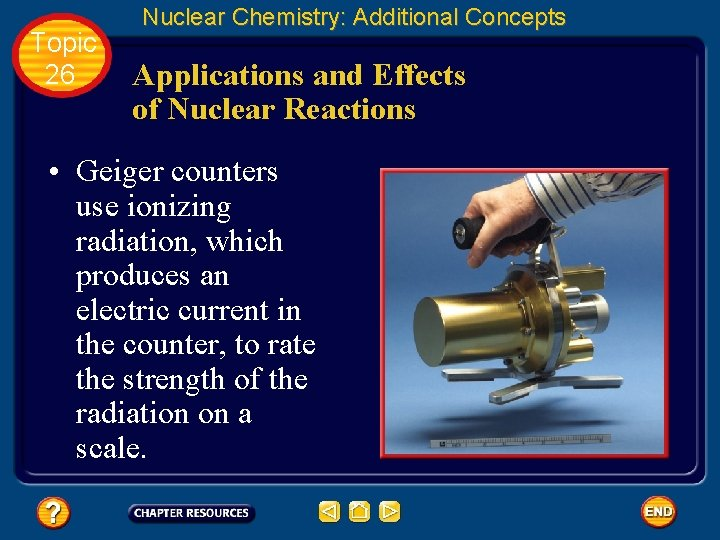Topic 26 Nuclear Chemistry: Additional Concepts Applications and Effects of Nuclear Reactions • Geiger counters use ionizing radiation, which produces an electric current in the counter, to rate the strength of the radiation on a scale.Topic 26 Nuclear Chemistry: Additional Concepts Applications and Effects of Nuclear Reactions • Ionizing radiation is radiation that is energetic enough to ionize matter upon collision.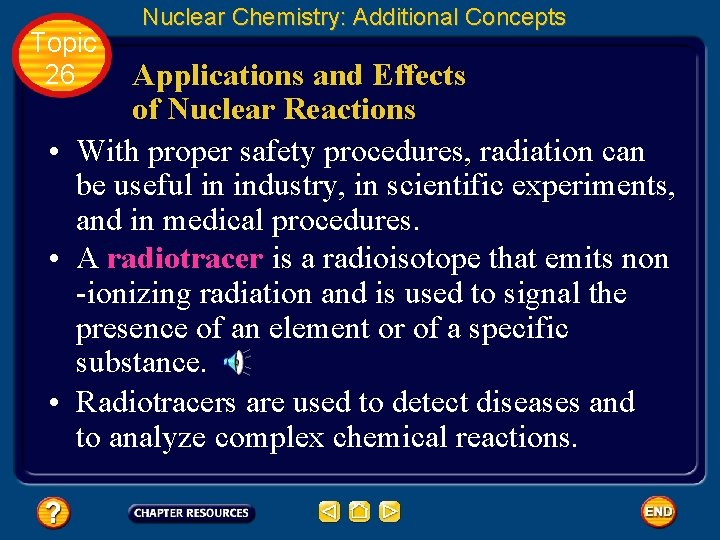Topic 26 Nuclear Chemistry: Additional Concepts Applications and Effects of Nuclear Reactions • With proper safety procedures, radiation can be useful in industry, in scientific experiments, and in medical procedures. • A radiotracer is a radioisotope that emits non -ionizing radiation and is used to signal the presence of an element or of a specific substance. • Radiotracers are used to detect diseases and to analyze complex chemical reactions.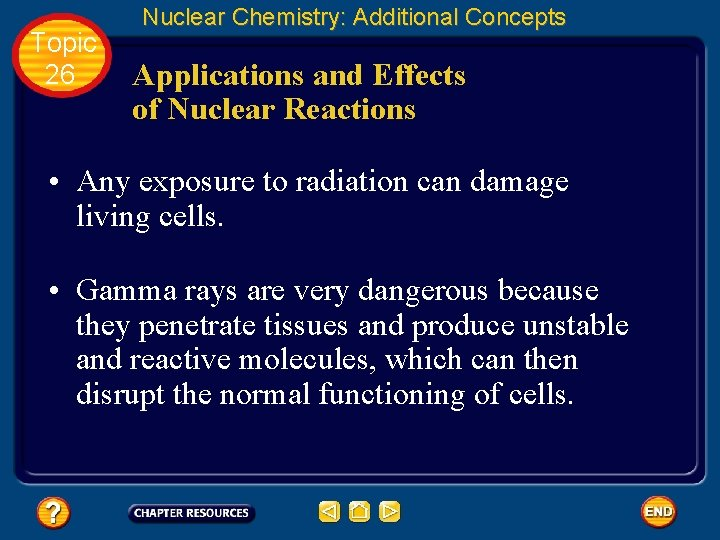Topic 26 Nuclear Chemistry: Additional Concepts Applications and Effects of Nuclear Reactions • Any exposure to radiation can damage living cells. • Gamma rays are very dangerous because they penetrate tissues and produce unstable and reactive molecules, which can then disrupt the normal functioning of cells.Topic 26 Nuclear Chemistry: Additional Concepts Applications and Effects of Nuclear Reactions • The amount of radiation the body absorbs (a dose) is measured in units called rads and rems. • Everyone is exposed to radiation, on average 100– 300 millirems per year. A dose exceeding 500 rem can be fatal.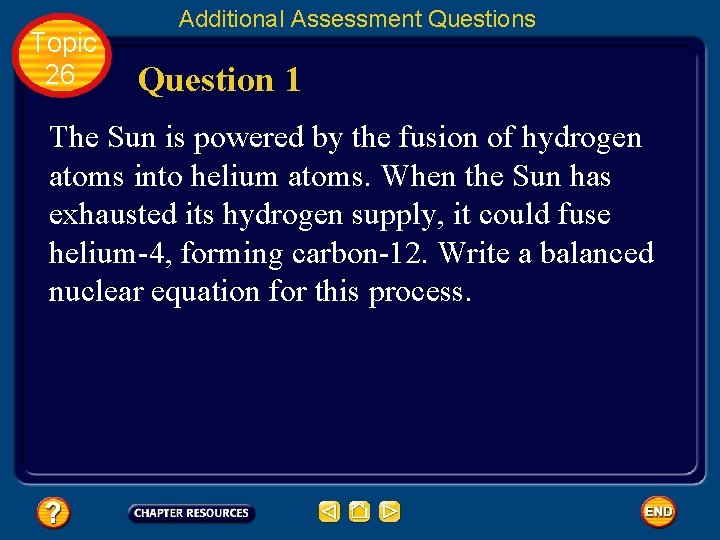Topic 26 Additional Assessment Questions Question 1 The Sun is powered by the fusion of hydrogen atoms into helium atoms. When the Sun has exhausted its hydrogen supply, it could fuse helium-4, forming carbon-12. Write a balanced nuclear equation for this process.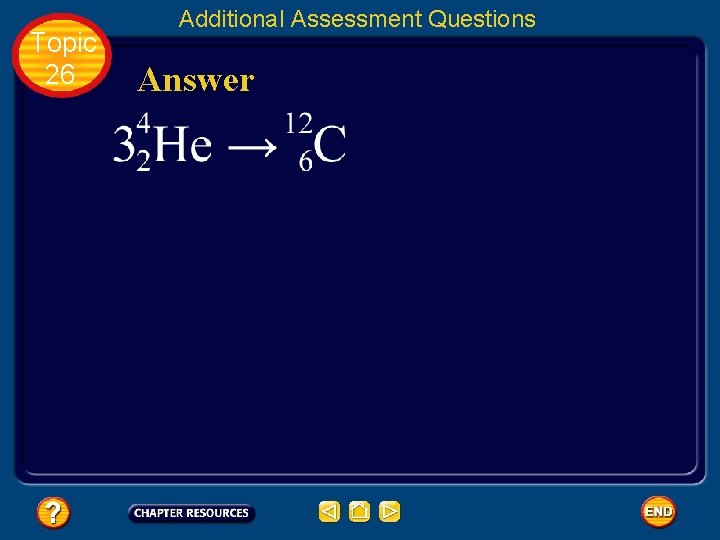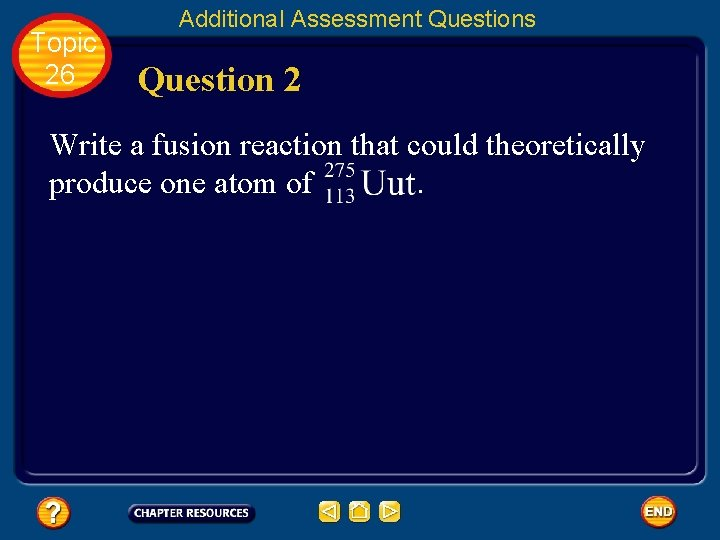Topic 26 Additional Assessment Questions Question 2 Write a fusion reaction that could theoretically produce one atom of .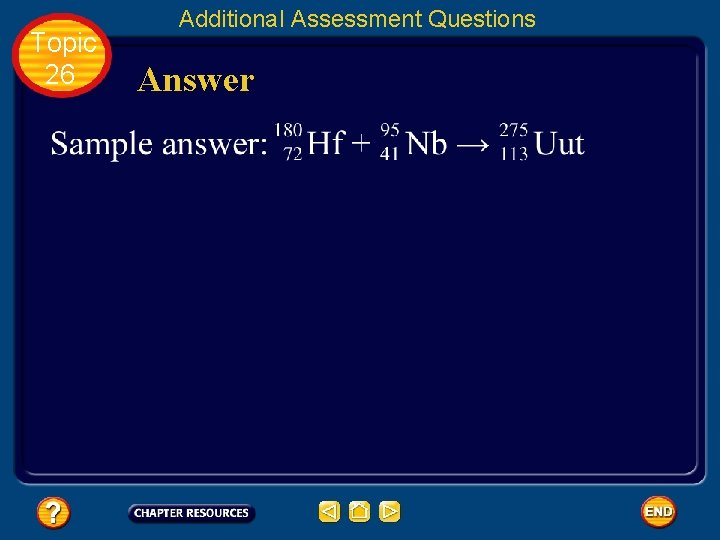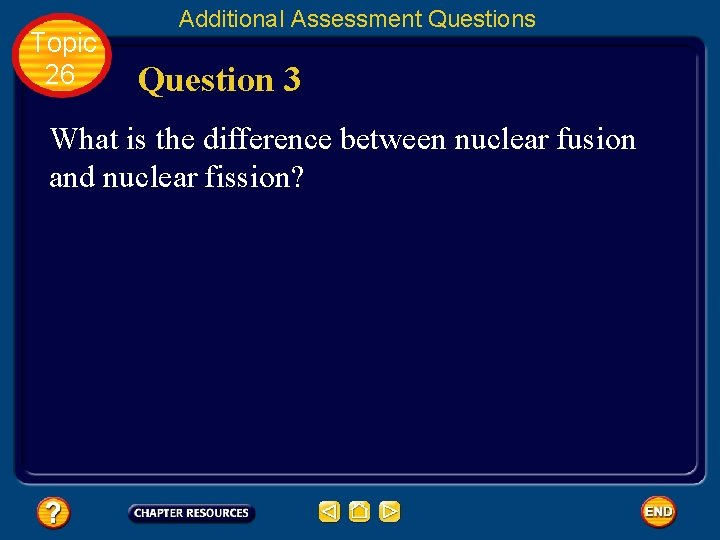Topic 26 Additional Assessment Questions Question 3 What is the difference between nuclear fusion and nuclear fission?Topic 26 Additional Assessment Questions Answer Nuclear fusion is the combining of nuclei to form a single nucleus. Nuclear fission is the splitting of a nucleus into fragments.Help To advance to the next item or next page click on any of the following keys: mouse, space bar, enter, down or forward arrow. Click on this icon to return to the table of contents Click on this icon to return to the previous slide Click on this icon to move to the next slide Click on this icon to open the resources file. Click on this icon to go to the end of the presentation.End of Topic Summary File